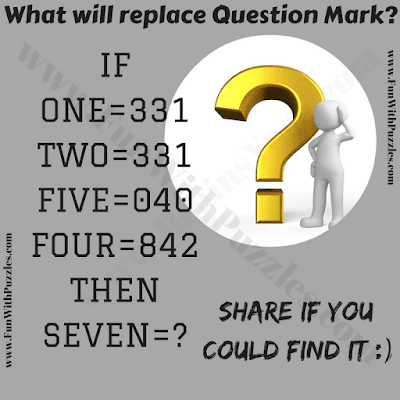Here the tough intelligence test question. In this tricky puzzle question, there are letters and numbers equations. Given letters equates to numbers on the right side of the equation. Can you find the logic used in the intelligence test question and then solve the last equation to find value of missing number which will replaces question mark?Can you solve this intelligence test question?

Answer of this "Intelligence Test Question", can be viewed by clicking on button. Please do give your best try before looking at the answer.